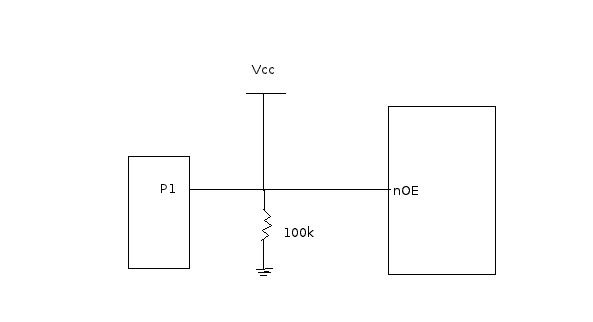# Confusion regarding pulldown resistors.

Hi,
I am having some confusion regarding pull down resistors. In the schematic I have a 100k pulldown resistor. P1 is the output port and the nOE(OE bar) is the input to a buffer.

What is confusing me is if the P1 input is high impedance then the nOE should go high, but when the P1 pin goes low isn't the nOE stuck again at high as the Vcc is never cutoff?vk6kro
Yes, that circuit is wrong. The supply is considered able to supply enough current to hold the input high no matter what the size of resistor you used for a pulldown.

You might use a pulldown where driven IC had an open circuit gate as an input. You would put in a pulldown to establish a LOW input even if the device was not being driven at that gate.

Then if the device was driven, the drive would determine the actual input since the pulldown would be a large resistance and its small current would not matter.

Otherwise, you could get random logic where the input could get static charges and change state.

But what if I need to put the logic to high and drive it low for enabling. Its a active low. So I need to drive it low to enable it.

vk6kro
But what if I need to put the logic to high and drive it low for enabling. Its a active low. So I need to drive it low to enable it.

Then you would use a pull-up resistor.

You only use pull down or pull up resistors when there is a need for them. If the gate is being driven by another gate's output, there is no need for either type of resistor. The gate output will be high or low and there will be no problem with the gate input.

Often, though, a gate may be driven by a switch or some sort of sensor that requires a resistor in the circuit to work.
A switch is a case where a pull down resistor might be needed. If you switch +5 volts to the input of a gate, and the gate input is very high impedance like CMOS, then the gate may acquire a positive charge and stay positive even if the switch is turned off. The pull-down resistor discharges any remaining charge on the gate and lets the gate go low.

Last edited:
sophiecentaur
Gold Member
It is worth pointing out that any input that is 'uncommitted' should be either held up or down by an appropriate resistor. CMOS circuits can get very upset if a gate voltage drifts to an undetermined 'half way' value.

If there is a pullup resistor and when I set the micrcontroller output to 0 then the output pin of the microcontroller (P1) will be set to ground and all the current should flow through the microcontroller output pin ground right?

vk6kro
If there is a pullup resistor and when I set the micrcontroller output to 0 then the output pin of the microcontroller (P1) will be set to ground and all the current should flow through the microcontroller output pin ground right?

Yes, that is right. That is called "sinking". Like water running down a sink.

So, you must make sure the resistor is large enough that the current into the micro is not too much for the micro outputs.

So, if the supply was 5 volts and the micro could sink 20 mA, then the pullup resistor would have to be more than 250 ohms. (5 volts / 0.02 amps = 250 ohms).

A common pullup resistor size would be 4.7 K.

Doubts cleared. Thanks for the clear explanations. :)